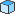# Altova FlowForce Server 2023 Advanced Edition

## Operators

To build FlowForce expressions, you can use the operators listed below. Remember that you can test any expression by calling the built-in functionsystem/compute.

Operator

Description

Example

==

Checks if a and b are equal (numerically equal for numbers, code-point equal for strings).

== 5 computes to true

2 + 3 == 4 computes to false

!=

Checks if a and b are not equal. Note that the following three expressions are equivalent:

a != b

not (a == b)

a <> b

+ 2 != 5 computes to true

+ 2 != 5 computes to false

<

Checks if a is less than b (numerically less for numbers, see below for strings).

computes to true

<=

Checks if a is less than or equal to b.

5 <=  computes to true

>

Checks if a is greater than b.

5 > 1  computes to true

>=

Checks if a is greater than or equal to b.

5 >=  computes to true

+

1 computes to 2

-

Subtraction.

1 computes to 1

*

Multiplication.

3 *  computes to 6

/

Division.

6 / 3 computes to 2

String comparisons are performed as follows:

The common prefix of the two strings are ignored (evaluated on code points)

If both remaining strings are non-empty, their first code points are compared numerically

Empty strings are less than non-empty strings

Use parentheses to instruct FlowForce to evaluate the expression inside first. For example:

2 + 3 * 4 computes to 14.

(2 + 3) * 4 computes to 20.